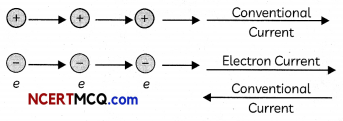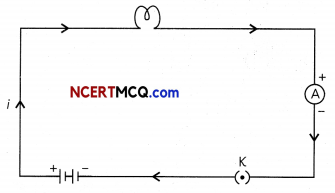## Electric Current and Circuit

Electricity is a form of energy and can be readily transmitted over large distances with relatively small loss in energy. In this chapter we will discuss about electric circuits, potential difference and flow of current in an electric circuit. We will also discuss Ohm’s law and the heating effect of electric current and its applications.

A continuous and closed path of an electric current is called an electric circuit.

## Electric Current

Electric current is expressed by the amount of charge flowing through a particular of area in unit time. It is defined as the rate of flow electric charges i.e., the charge flowing per unit time.

Let Q denote the amount of charge flowing across a given point in the conductor in time t, then the current flowing is given by, I = Q/t.

## Conventional Direction of Current flow

It is the direction in which the positive charges flow which is opposite to the direction of the flow of negative charges.Ampere: One Ampere is defined as the current flowing when 1 Coulomb of charge flows across a point in 1 second.
1 Ampere = $$\frac{1 \text { Coulomb }}{1 \text { second }}$$

Small quantities of current are expressed in milliampere (1 mA = 10-3 A) or in microampere (1 yA = 10-6A).

Coulomb: It is the SI unit of charge and is the charge carried by 6 × 1018 electrons, since the charge of 1 electron = 1.6 × 10-19 C.Example 1.
Calculate the number of electrons constituting one coulomb of charge.
n = $$\frac{q}{e}=\frac{1}{1.6 \times 10^{-19}}$$ = 6.25 × 1018 electrons